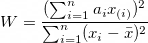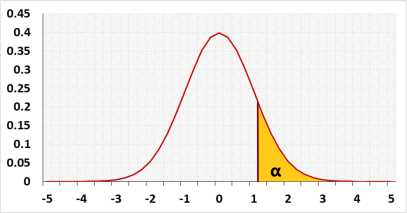# Shapiro-Wilk Test Calculator

*The maximum sample size is 5000

Video Shapiro Wilk Test Information
When entering data, press comma , , Space or Enter after each value.
You may copy and paste data from Excel or Google Sheets. Leaving empty cells is okay. The tool doesn't count empty cells or non-numeric cells.

## Information

Target: To check if the normal distribution model fits the observations
The tool combines the following methods:
1. A formal normality test: Shapiro-Wilk test, this is one of the most powerful normality tests.
2. Graphical methods: QQ-Plot chart and Histogram.

The Shapiro Wilk test uses only the right-tailed test. When performing the test, the W statistic is only positive and represents the difference between the estimated model and the observations. The bigger the statistic, the more likely the model is not correct. The left-tailed may represent a value that is too small, the W statistic can't be too small.

Small sample size (n ≤ 50)
When the sample size: the tool calculates the p-value from the exact tables, with the following p-values: 0.01 0.02 0.05 0.1 0.5 0.9 0.95 0.98 0.99. usually, W value will be between two cells, and the p-value calculation will be done as a harmonic interpolation between the two p-values. The p-values is very accurate around the common significance levels.
Compare to other test the Shapiro Wilk has a good power to reject the normality, but as any other test it need to have sufficient sample size, around 20 depend on the distribution, see examples

In this case the normal distribution chart is only for illustration.

Large sample size (n > 50)
The tool uses the normal approximation. Since the sample size is large the approximation is good for any p-value.

* The maximum sample size is 5000, but since no distribution is exactly normal distribution, a very large sample size has the power to reject the normality assumption for almost any distribution even if the difference from the normal distribution is minimal.

Hypotheses
H0: Normal distribution
H1: Other distribution
Test statisticNormal distribution## R Code

The following R code should produce the same results:

Since the tool and R uses approximations, the p-value results are not always identical. R uses the normal approximations for n≥12 and a different approximation for smaller samples, while the tool uses the normal approximation for n≥50.